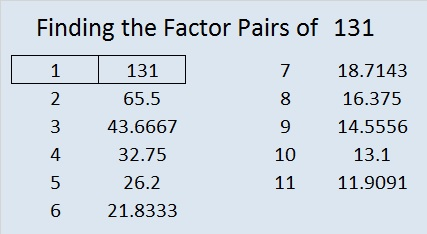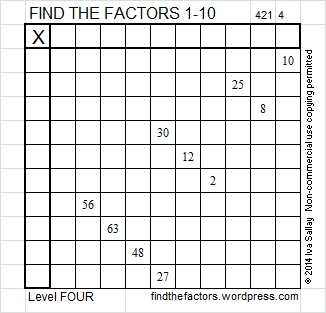# 131 and Level 4

• 131 is a prime number.
• Prime factorization: 131 is prime.
• The exponent of prime number 131 is 1. Adding 1 to that exponent we get (1 + 1) = 2. Therefore 131 has exactly 2 factors.
• Factors of 131: 1, 131
• Factor pairs: 131 = 1 x 131
• 131 has no square factors that allow its square root to be simplified. √131 ≈ 11.4455How do we know that 131 is a prime number? If 131 were not a prime number, then it would be divisible by at least one prime number less than or equal to √131 ≈ 11.4. Since 131 cannot be divided evenly by 2, 3, 5, 7, or 11, we know that 131 is a prime number.

131 is never a clue in the FIND THE FACTORS puzzles.Excel file of puzzles and previous week’s solutions: 10 Factors 2014-05-261.Joseph Nebus
2.ivasallay